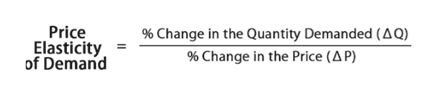# After a careful statistical analysis, the Chidester Company concludes the demand function for its product isQ = 500 - 3P + 2Pr + 0.1Iwhere Q is the quantity demanded of its product, P is the price of its product, Pr is the price of its rival’s product, and I is per capita disposable income (in dollars). At present, P = \$10, Pr = \$20, and I = \$6,000. What is the cross- price elasticity of demand between its product and its rival’s product?  a.0.0388  b.0.0721  c.0.0360 d.-0.0360

Question
27 views

After a careful statistical analysis, the Chidester Company concludes the demand function for its product is

= 500 - 3+ 2Pr + 0.1I

where is the quantity demanded of its product, is the price of its product, Pr is the price of its rival’s product, and is per capita disposable income (in dollars). At present, = \$10, Pr = \$20, and = \$6,000.

What is the cross- price elasticity of demand between its product and its rival’s product?

 a. 0.0388 b. 0.0721 c. 0.036 d. -0.036
check_circle

Step 1

The formula of Price Elasticity...help_outlineImage TranscriptionclosePrice Elasticity of Demand % Change in the Quantity Demanded (AQ) % Change in the Price (A P) fullscreen

### Want to see the full answer?

See Solution

#### Want to see this answer and more?

Solutions are written by subject experts who are available 24/7. Questions are typically answered within 1 hour.*

See Solution
*Response times may vary by subject and question.
Tagged in